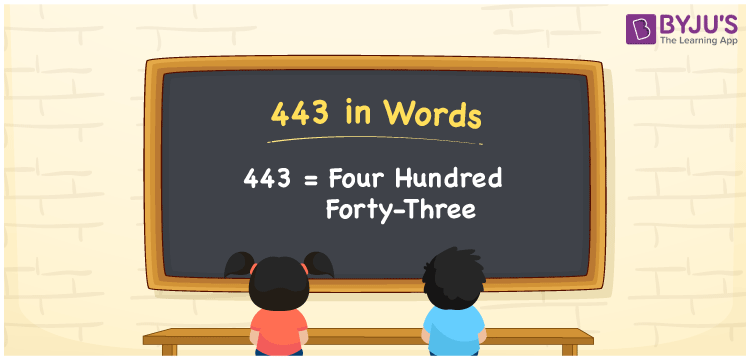# 443 in Words

We can write 443 in words as Four hundred forty-three. If the price of a toy is Rs. 443, then you can say, “The price of the toy is Four hundred forty-three rupees”. Here, Four hundred forty-three is the number name of 443. In this article, you will learn how to write the number 443 in words using a place value chart, along with some interesting facts about 443.

 443 in words Four hundred forty-three Four hundred forty-three in Numbers 443

## 443 in English Words

We generally use the English alphabet to express numbers in words. Thus, we spell 443 in English words as “Four hundred forty-three”.## How to Write 443 in Words?

Below is the place value chart for the three-digit number, i.e., 443. From this table, we can convert the number 443 into words.

 Hundreds Tens Ones 4 4 3

Here, ones = 3, tens = 4, hundreds = 4

By expanding the above digits, we get;

4 × Hundred + 4 × Ten + 3 × One

= 4 × 100 + 4 × 10 + 3 × 1

= 400 + 40 + 3

= Four hundred + Forty + Three

= Four hundred forty-three

Therefore, 443 in words = Four hundred forty-three

We know that 443 is a natural number that precedes 444 and succeeds 442.

443 in words – Four hundred forty-three

Is 443 an even number? – No

Is 443 an odd number? – Yes

Is 443 a prime number? – Yes

Is 443 a composite number? – No

Is 443 a perfect square number? – No

Is 443 a perfect cube number? – No

## Frequently Asked Questions on 443 in Words

Q1

### How do you spell 443?

We can spell the number 443 in English words as Four hundred forty-three.
Q2

### Write the value of 1 + 443 in words.

1 + 443 = 444 Therefore, the value of 1 + 443, i.e. 444 in words Four hundred forty-four.
Q3

### How to write Rs. 443 in words on a cheque?

On a cheque, we can write Rs. 443 in words as “Four hundred forty-three rupees only”.